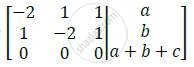Advertisement Remove all ads

# Show that the Following Equations: -2x + Y + Z = A, X - 2y + Z = B, X + Y - 2z = C Have No Solutions Unless a +B + C = 0 in Which Case They Have Infinitely Many Solutions. Fin - Applied Mathematics 1

Show that the following equations: -2x + y + z = a, x - 2y + z = b, x + y - 2z = c have no solutions unless a +b + c = 0 in which case they have infinitely many solutions. Find these solutions when a=1, b=1, c=-2.

Advertisement Remove all ads

#### Solution

Part I:
-2x + y + z = a
x - 2y + z = b
x + y - 2z = c
Writing the equations in the matrix form,

[[-2,1,1],[1,-2,1],[1,1,-2]].[[x],[y],[z]]=[[a],[b],[a+b+c]]

Augmented matrix [A|B]=Number of unknowns = n = 3
Rank of A (rA) = Number of non-zero rows in A = 2

Case I: No Solution
For which, rA < rAB
This is only possible, when ‘a+b+c≠0’ upon which,
Rank of [A|B] = (rAB) = 3

Case II: Infinite Solution
For which, rA = rAB < n (i.e. < 3)
This is only possible, when ‘a+b+c=0’ upon which,
Rank of [A|B] = rAB = 2

Part II: Put a= 1, b = 1, c = -2, in (1)

[[-2,1,1],[1,-2,1],[0,0,0]].[[x],[y],[z]]=[,,]

R^2 – R^1; → [[-2,1,1],[3,-3,0],[0,0,0]].[[x],[y],[z]]=[,,]                        …(2)

Here, n – rA = 3 – 2 = 1
We have to assume one unknown.
Let y = t (≠0)
On expanding (2), 3x – 3y = 0
∴ x – y = 0
∴ x = y = t
And, -2x + y + z = 1
∴ -2t + t + z = 1
∴z = 1 + t
Hence, the solution is x = t, y = t, z = 1 + t (Infinite Solution)

Concept: Rank of a Matrix Using Echelon Forms
Is there an error in this question or solution?
Advertisement Remove all ads

#### APPEARS IN

Advertisement Remove all ads
Advertisement Remove all ads
Share
Notifications

View all notifications

Forgot password?